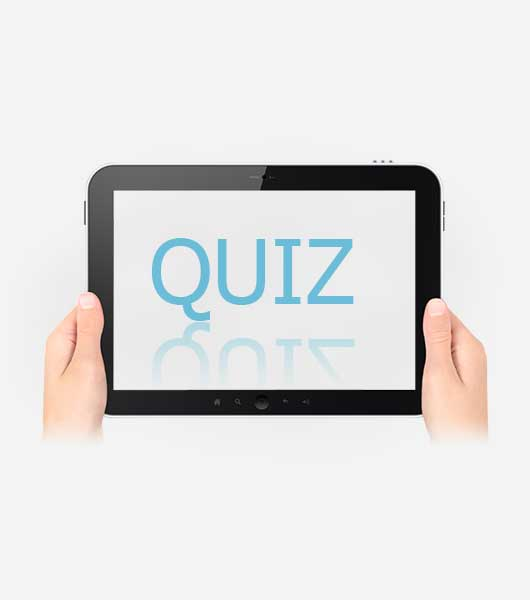# Ssab Six Sigma Green Belt Knowledge Quiz

55 Questions | Attempts: 118
ShareSettings100 minute timed quiz to answer 52 required questions and three optional bonus questions. There is no penalty for answering the bonus questions and you must complete the quiz once you start it. All questions are worth one point. You will be allowed three attempts to score 80% or higher to pass the knowledge test. Good luck!

• 1.
In the process map below which terms best describe the labeled items (a, b, c) of the process map?
• A.

A=process step; b=rework-loop; c= transport

• B.

A=storage; b=decision point; c=process step

• C.

A=proces step; b=decision point; c=rework loop

• D.

A=measurement point; b=transport; c=rework loop

• 2.
Average moving range is the preferred statistic over standard deviation when evaluating the dispersion of a data set.
• A.

True

• B.

False

• 3.
Which of the following is a "red flag" for considering not going forward with a project?
• A.

The project has already been done

• B.

The project will not show savings

• C.

The project will have a negative impact on another area

• D.

All of the above

• E.

A & c

• 4.
When at all possible, you should randomize the runs of your data collection for measurement system analysis.
• A.

True

• B.

False

• 5.
One concern with attribute data is that it typically requires three to five times as much data per data set than continuous data when drawing an inference about the data set.
• A.

True

• B.

False

• 6.
Which of the following is not a preferred method for selecting a project? (Choose best answer)
• A.

• B.

Choosing a project that aligns with departmental goals

• C.

Soliciting vendor solutions to problems

• D.

Having the superintendent suggest a single solution to the problem

• E.

C&d

• 7.
In the Pareto chart below, the black dotted line represents what?
• A.

Cumulative percent of the data

• B.

Goal of the project

• 8.
Minitab worksheets function in the same way and are formatted in the exact same way as an excel worksheet?
• A.

True

• B.

False

• 9.
Relative to MSAs, the "Rs" in Gauge R&R stand for repeatability and reproducibility.
• A.

True

• B.

False

• 10.
Continuous and variable data are synonymous like discrete and attribute data are synonymous?
• A.

True

• B.

False

• 11.
On a control chart such as an I-MR what determines the upper and lower control limits of the two different charts)
• A.

The customer

• B.

The process owner

• C.

• D.

The process data

• 12.
The value for R-squared indicates how well the reqression equation for a given set of data "fits" the data or the accuracy of the regression model (check one)
• A.

True

• B.

False

• 13.
This high level mapping tool in the form of a matrix and a three step process map is used to show a process in a systematic breakdown of each step from supplier to customer:
• A.

Detailed process map

• B.

Value stream map

• C.

SIPOC

• D.

C&E diagram

• 14.
In hypothesis testing, a standard or default p-value evaluation limit (alpha risk) is set for rejecting or failing to rejecting the null hypothesis.  If the p-value is less than typical value of  _____, then reject the null?
• A.

0.05

• B.

0.00005

• 15.
If the alpha risk (α) for a process is selected to be 0.10, then the P-value must be less than _____ to reject the null
• A.

0.05

• B.

0.10

• C.

1.00

• D.

5.00

• 16.
If the process owner chooses to reduce the alpha risk (α) for a process to decrease the liabililty to the customer, then sample sizes for inferential statistics must increase or decrease: (choose one)
• A.

Decrease

• B.

Increase

• 17.
What is the main purpose of a C&E matrix:
• A.

To create a process map

• B.

To use the knowledge of the group to determine the critical few (x’s)

• C.

To find the hidden factory

• D.

To test for correlation

• 18.
In the following control chart, the red squares with the number "1" above them represent what?  Check all that apply.
• A.

Outliers or out of control points

• B.

Bad data that should automatically be thrown out

• C.

Nine successive points in the same direction

• 19.
Six Sigma derives its name from +3 and -3 standard deviation above and below the mean of a standard normal distribution.
• A.

True

• B.

False

• 20.
What is an example of mistake proofing?
• A.

Adding a flashing light as a visual indicator

• B.

5S event of an area

• C.

Using a color coding system

• D.

All of the above

• 21.
Normal and Non-Normal data types have no effect on the performace metrics in Process Capability analysis
• A.

True

• B.

False

• 22.
The term "Poka-Yoke" is also know as...?
• A.

Mistake proofing or errors to avoid

• B.

Hoshin kanri

• C.

Muda

• D.

The first step in 5S

• 23.
The C&E in a fishbone diagram is short for what?
• A.

Cause and effect

• B.

Cost and effort

• C.

Customer and employee

• D.

None of the above

• 24.
Special Cause:  Variation that is characterized by a pattern of variation that changes over time and may be show as outlier, drift, and shift in a process.
• A.

True

• B.

False

• 25.
Process capability shows the ratio of the Voice of the Process (VOP) to the Voice of the Customer (VOC)?
• A.

True

• B.

False

## Related TopicsBack to top